• 2021-08-25 19:10:23

## 箱型图查找异常值并替换

	定义为值大于上四分位或者小于下四分位的值位异常值，若是异常值，则用mean替换，项目中遇到的，但是这个可能或将0值也替换了，但是0值不是异常值，故而舍弃这种方法。

import numpy as np
import pandas as pd
import matplotlib.pyplot as plt
from scipy import stats

'''
异常值分析
'''

# 加载数据

def get_data(df, row):
# 截取第一列的数据
df = df.iloc[:, 1]
# 返回的是一个series，获取第一个序列
'''
1   {}
2   {}
.
.
.
10  {}
'''
series_1 = df[row]
# 返回的序列用逗号将其split，返回的是list类型，每个元素都是str，要将其转换成float，方便后边计算上下四分位
list_1 = series_1.split(',')
# 返回的是list类型，每个元素都是str，要将其转换成float，方便后边计算上下四分位
for i in range(len(list_1)):
list_1[i] = float(list_1[i])

# 将list转换成series，因为box_plot_outliers接受的参数是series类型
series = pd.Series(list_1)
print(series)
return series

def box_plot_outliers(data_ser, box_scale):
'''
利用箱型图去除异常值
:param data_ser: 接收pandas.Series数据格式
:param box_scale: 箱型图尺寸，取3
:return:
'''
print(box_scale)
print(type(box_scale))
s = (data_ser.quantile(0.75) - data_ser.quantile(0.25))
print(s)
print(type(s))
iqr = box_scale * (data_ser.quantile(0.75) - data_ser.quantile(0.25))
# 下阈值
val_low = data_ser.quantile(0.25) - iqr * 0.5
# 上阈值
val_up = data_ser.quantile(0.25) + iqr * 0.5
# 异常值
outlier = data_ser[(data_ser < val_low) | (data_ser > val_up)]
# 正常值
normal_val = data_ser[(data_ser > val_low) & (data_ser < val_up)]
return outlier, normal_val, val_low, val_up

if __name__ == '__main__':
series = get_data(df, 2)
outlier, normal_val, val_low, val_up = box_plot_outliers(series, 3)
print('异常值:' + str(outlier))
outlier = np.array(outlier)
print('val_up的类型：{}'.format(type(val_up)))
print('normal_val的类型:{}'.format(type(normal_val)))
print(normal_val)
sum = 0  # 累加非异常值的数
normal_val = np.array(normal_val)
for i in range(len(normal_val)):
sum += normal_val[i]
# 刨除异常值后的均值，因为异常值可能对均值的影响很大
mean = sum / (len(normal_val))
print('均值：{}'.format(mean))
if outlier is None:
print('没有异常值')
else:
for i, val in enumerate(series):
# 比如【pandas.mean()求平均值后是一个<class 'numpy.float64'>类型】转换成Python的float类型numpy.float(value)
val_up = np.float(val_up)
val_low = np.float(val_low)
# 比较大小，如果在大于上四分位或者小于下四分位，则将其替换为位mean，如果不是则采用真实值val
series[i] = float(np.where((((val < val_low) | (val > val_up))), mean, val))
print(series)
print(series)python
更多相关内容
• 我就废话不多说了，直接上代码吧！ # -*- coding: utf-8 -*- import pandas as pd import matplotlib.pyplot as plt catering_sale = '../data/catering_sale.xls' data = pd.read_excel(catering_sale, index_col=...
• 在做数据分析时，我们免不了要检查数据中的异常值，但是什么样的数据算作异常呢。有人说很大或者很小的值，那到底多大多小的值算异常。箱型图就可以很好的解决这个问题。我们都知道折线图、柱状图等，但很少使用过...在做数据分析时，我们免不了要检查数据中的异常值，但是什么样的数据算作异常呢。有人说很大或者很小的值，那到底多大多小的值算异常。箱型图就可以很好的解决这个问题。我们都知道折线图、柱状图等，但很少使用过箱型图。关于箱型图，百度百科的解释为：

箱形图（Box-plot）又称为盒须图、盒式图或箱线图，是一种用作显示一组数据分散情况资料的统计图。因形状如箱子而得名。在各种领域也经常被使用，常见于品质管理。它主要用于反映原始数据分布的特征，还可以进行多组数据分布特征的比 较。箱线图的绘制方法是：先找出一组数据的最大值、最小值、中位数和两个四分位数；然后， 连接两个四分位数画出箱子；再将最大值和最小值与箱子相连接，中位数在箱子中间。

通过定义可以看出，箱型图可以很好的展示数据的基本分布情况，而且可以很方便的检测出数据中的异常值。

我们先介绍两种箱型图的实现方式，再详解箱型图含义，最后结合Kaggle数据集看看如何使用。

# 1.pandas实现

import matplotlib.pyplot as plt
import numpy as np
import pandas as pd

df = np.random.randn(3,4)
df = pd.DataFrame(df)
df.boxplot()

这里用的pandas自带的绘图方法，其实就是matplotlib。df的数据内容为：

	    0            1	        2	        3
0	-0.803730	2.666505	1.668437	-2.542154
1	0.996711	0.532948	1.484964	-0.452487
2	-1.036156	0.127020	-0.381138	-0.688037

得到的箱型图如下：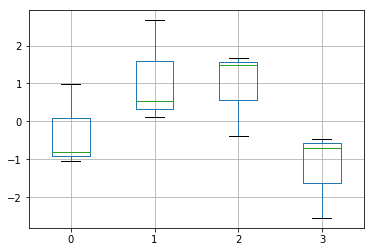# 2.matplotlib实现

import matplotlib.pyplot as plt
import numpy as np

all_data = [np.random.normal(0, std, 100) for std in range(1, 4)]

fig = plt.figure(figsize=(8,6))

plt.boxplot(all_data,
notch=False, # 是否用盒子形状
sym='rs',    # 用红色矩形展示异常值
showmeans=True,#展示均值点
patch_artist=False,#是否要填充色
meanline=False,#展示均值线
widths=0.5,#设置箱盒宽度
vert=True)   #垂直展示图形

plt.xticks([y+1 for y in range(len(all_data))], ['x1', 'x2', 'x3'])
plt.xlabel('measurement x')
t = plt.title('Box plot')
plt.show()

图形如下：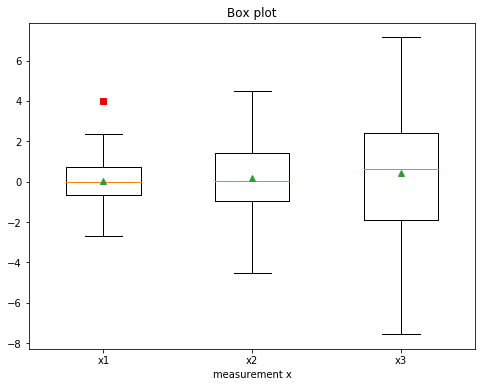水平展示的效果如下：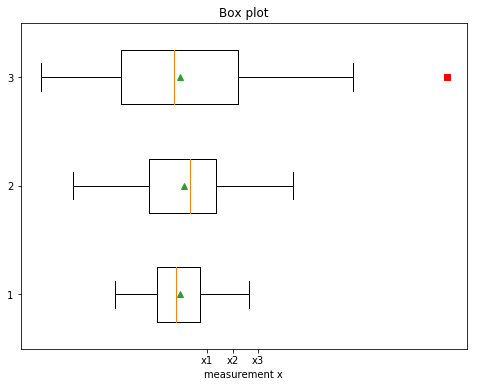# 3.图形详解

我们结合例子来讲解图形所代表的意思，例子来源于MBA智库百科，链接在文末。我们有如下数据：

2710 2755 2850 | 2880 2880 2890 | 2920 2940 2950 | 3050 3130 3325

计算Q1，Q2，Q3，

Q1 = (2850 + 2880) / 2 = 2865

Q2 = (2890 + 2920) / 2 = 2905

Q3 = (2950 + 3050) / 2 = 3000

也就是说，中位数是2905，第一个四分位数（也叫下四分位数）Q1 = 2865，第三个四分位数（也叫上四分位数）Q3 = 3000。有了四分位数Q1和Q3，可以算出四分位数全距IQR = Q3 − Q1。从而得到下限和上限。在上下限之外的点就是异常点。如下图所示：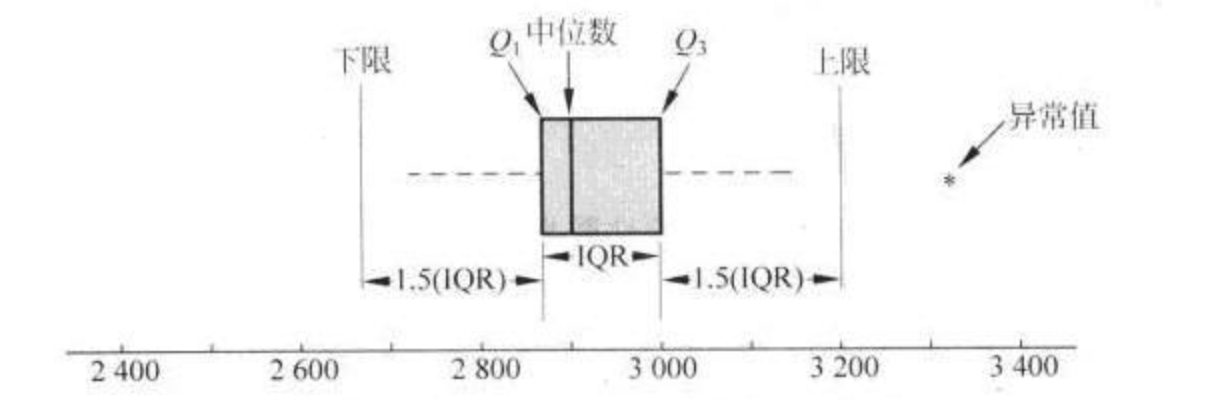# 4.实际应用

我们通过箱型图来检测Kaggle数据集Give Me Some Credits中的异常值。先看代码：

import pandas as pd
import matplotlib.pyplot as plt

#查看数据集中的如下几列数据
data = data[['NumberOfTime30-59DaysPastDueNotWorse','NumberOfTimes90DaysLate','NumberOfTime60-89DaysPastDueNotWorse','age']]

#使用pandas绘制
plt.figure(figsize=(10,5))
data.boxplot(sym='r*',vert=False,patch_artist=True,meanline=False,showmeans=True)
plt.show()

得到图形：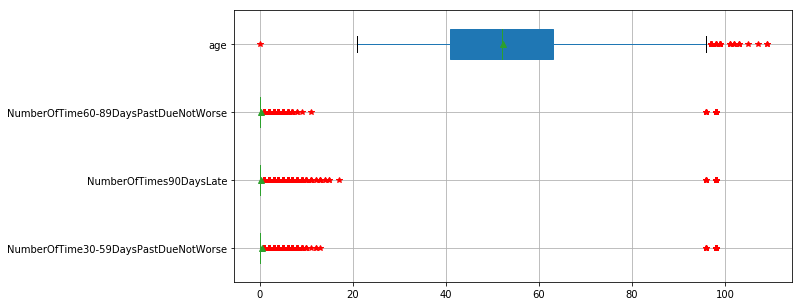我们可以看出，age的取值有一个明显的异常点0。另外三列也有明显的异常点，97和98。对于这样的异常值直接删除即可。是不是很方便、很直观。箱型图简直就是数据分析中的异常值检测的神器。

参考资料：

https://matplotlib.org/gallery/pyplots/boxplot_demo_pyplot.html#sphx-glr-gallery-pyplots-boxplot-demo-pyplot-py

http://blog.topspeedsnail.com/archives/737

https://wiki.mbalib.com/wiki/%E7%AE%B1%E7%BA%BF%E5%9B%BE

展开全文Python
• 异常值检测的方法有很多，通过数据分布图型寻找异常值、算法模型（聚类、随机森林等），我这里就是记录一下工作中做初步的数据探查时用到的箱型图检测和正态分布检测，这两种都是根据数据分布情况来识别异常值的，...

异常值检测的方法有很多，通过数据分布图型寻找异常值、算法模型（聚类、随机森林等），我这里就是记录一下工作中做初步的数据探查时用到的箱型图检测和正态分布检测，这两种都是根据数据分布情况来识别异常值的，没有结合到业务的层面，在做初步的探查时还是高效且适用的。因为正态分布3σ的异常值检测需要数据符合正态分布，现实情况中大部分数据都是杂乱无章的，因此重点使用的是箱型图检测。

1.箱型图的优势

（1）准确稳定地描绘出数据的离散分布情况且不需要服从特定的分布形式

箱形图的绘制依靠实际数据，不需要事先假定数据服从特定的分布形式，没有对数据作任何限制性要求，它只是真实直观地表现数据形状的本来面貌；

（2）异常值不会影响四分位数的确定

箱形图判断异常值的标准以四分位数四分位距为基础，四分位数具有一定的耐抗性，多达25%的数据可以变得任意远而不会很大地扰动四分位数，所以异常值不能对这个标准施加影响，箱形图识别异常值的结果比较客观。

2.箱型图的示意及符号说明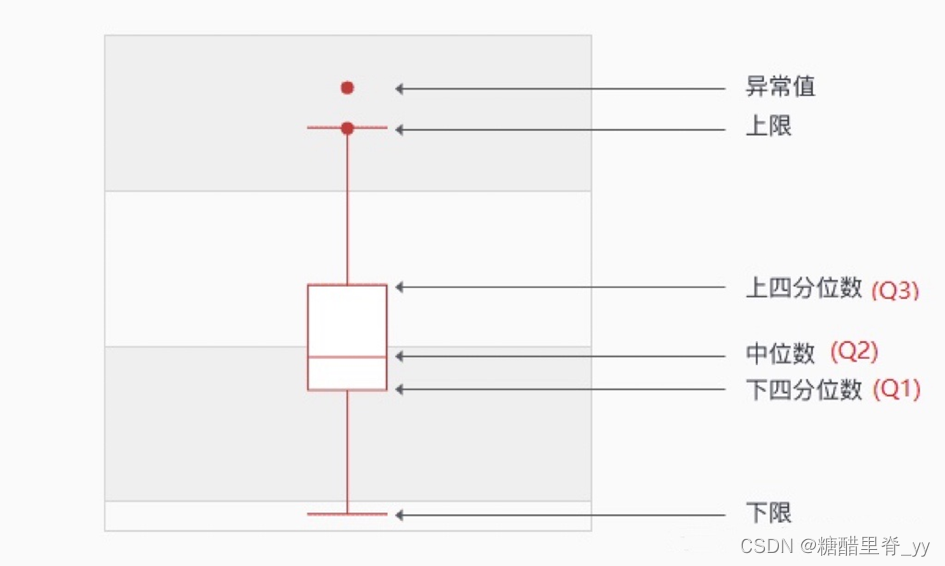（图片来源于网络）

Qi所在位置=i（n+1）/4，其中i=1，2，3。n表示序列中包含的项数。根据所在位置找到此位置上的数据。

下四分位数Q1：该样本中所有数值由小到大排列后第25%的数字。

中位数Q2：该样本中所有数值由小到大排列后第50%的数字

上四分位数Q3：该样本中所有数值由小到大排列后第75%的数字

四分位距IQR：IQR=Q3-Q1

上限：非异常范围内的最大值，上限=Q3+1.5IQR

下限：非异常范围内的最小值，下限=Q1-1.5IQR

3.Python代码

def OutlierDetection_box(df,con,path):#con代表数据列的名称，path代表文本保存的路径
# 计算下四分位数和上四分位
Q1 = df.quantile(q=0.25)
Q3 = df.quantile(q=0.75)
Q2 = df.quantile(q=0.5)

# 基于1.5倍的四分位差计算上下须对应的值
low_whisker = Q1 - 1.5 * (Q3 - Q1)
up_whisker = Q3 + 1.5 * (Q3 - Q1)

# 寻找异常点
outliers = df[(df > up_whisker) | (df < low_whisker)]
#data1 = pd.DataFrame({'id': kk.index, '异常值': kk})

#统计，stat_ll为箱型图中数据分布情况（多个数据列合并最后会输出一个sheet页），f最后会输出一个标识具体数据点的文本
dataQ3=df[(df>Q2) & (df<=Q3)]
countQ3=len(dataQ3)
dataQ1 = df[(df < Q2) & (df >= Q1)]
countQ1 = len(dataQ1)
data_max = df[(df <= up_whisker) & (df > Q3)]
count_max = len(data_max)
data_min = df[(df >= low_whisker) & (df < Q1)]
count_min = len(data_min)
stat_ll=[con,countQ3,countQ1,count_max,count_min]
with open(path+"/box_new.txt", "a",encoding='utf-8') as f:
f.write("\n%s的箱型图分布统计如下："%con)
f.write("\n箱线图检测到的异常值如下：")
f.write(str(outliers.to_dict()))
f.write("\n落入中位数到上四分位距有%d条，具体如下："%countQ3)
f.write(str(dataQ3.to_dict()))
f.write("\n落入中位数到下四分位距有%d条，具体如下：" % countQ1)
f.write(str(dataQ1.to_dict()))
f.write("\n落入上四分位数到最大值有%d条，具体如下：" % count_max)
f.write(str(data_max.to_dict()))
f.write("\n落入下四分位数到最小值有%d条，具体如下：" % count_min)
f.write(str(data_min.to_dict()))
return outliers,stat_ll

2.正态分布

def OutlierDetection_std(df,con,path):
# 计算均值
u = df.mean()
# 计算标准差
std = df.std()
# 计算P值
res = kstest(df, 'norm', (u, std))
if res <= 0.05:#判断是不是符合正态分布
# 定义3σ法则识别异常值
# 识别异常值
error_low = df[np.abs(df - u) > 2 * std]
error_high = df[np.abs(df - u) > 3 * std]
# 剔除异常值，保留正常的数据
#data_normal = df[np.abs(df - u) <= 3 * std]
with open(path+"/std_outliers.txt", "a", encoding='utf-8') as f:
f.write("\n%s符合正态分布："%con)
f.write("\n2sigma异常值如下：")
f.write(str(error_low.to_dict()))
f.write("\n3sigma异常值如下：")
f.write(str(error_high.to_dict()))
return u, std, error_low, error_high

else:
logger.info('该数据不服从正态分布-----------')
return None


3.正态分布结合四分位距（网上一篇文章看到的，实际没有使用）

def box_3Sigma(df,sigma):
Q1 = df.quantile(q=0.25)
Q3 = df.quantile(q=0.75)
IQR = Q3 - Q1
min = Q1 - 1.5 * IQR
max = Q3 + 1.5 * IQR
ratio = 0.3
low = 3*sigma * ratio + min * (1 - ratio)
hight = 3*sigma * ratio + max * (1 - ratio)

展开全文python
• 箱型图 通俗来讲，就是将所有的数据都分布在这张图上，矩形上下限用四分为值，又用四分位值算出一个上界和下界，大于上界或者小于下界的值就是异常值。这里四分位距（IQR)就是上四分位与下四分位的差值。我们通过...

## 一.箱型图

通俗来讲，就是将所有的数据都分布在这张图上，矩形上下限用四分为值，又用四分位值算出一个上界和下界，大于上界或者小于下界的值就是异常值。这里四分位距（IQR)就是上四分位与下四分位的差值。我们通过四分位距的1.5倍为标准，上界：超过上四分位+1.5倍IQR距离，下界：下四分位-1.5倍IQR距离的点为异常值。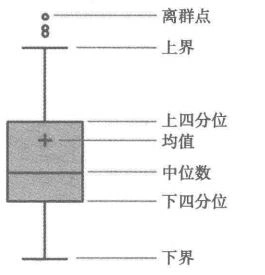分位值的定义和计算在这里:(https://blog.csdn.net/crj0926/article/details/100853490)

## 二.matplotlib模块

Matplotlib 是一个 Python 的 2D绘图库，通过 Matplotlib，pathon仅需要几行代码，便可以生成绘图，直方图，功率谱，条形图，错误图，散点图等。

## 三.构造箱型图代码

1. boxplot函数参数：

x：指定要绘制箱线图的数据；

notch：是否是凹口的形式展现箱线图，默认非凹口；

sym：指定异常点的形状，默认为+号显示；

vert：是否需要将箱线图垂直摆放，默认垂直摆放；

whis：指定上下须与上下四分位的距离，默认为1.5倍的四分位差；

positions：指定箱线图的位置，默认为[0,1,2…]；

widths：指定箱线图的宽度，默认为0.5；

patch_artist：是否填充箱体的颜色；

meanline：是否用线的形式表示均值，默认用点来表示；

showmeans：是否显示均值，默认不显示；

showcaps：是否显示箱线图顶端和末端的两条线，默认显示；

showbox：是否显示箱线图的箱体，默认显示；

showfliers：是否显示异常值，默认显示；

boxprops：设置箱体的属性，如边框色，填充色等；

labels：为箱线图添加标签，类似于图例的作用；

filerprops：设置异常值的属性，如异常点的形状、大小、填充色等；

medianprops：设置中位数的属性，如线的类型、粗细等；

meanprops：设置均值的属性，如点的大小、颜色等；

capprops：设置箱线图顶端和末端线条的属性，如颜色、粗细等；

whiskerprops：设置须的属性，如颜色、粗细、线的类型等；

2. 代码:

#导入模块
import pandas as pd
import matplotlib.pyplot as plt
# 导入数据
# 检查年龄是否有缺失
any(titanic.age.isnull())
# 删除含有缺失年龄的观察
titanic.dropna(subset=['age'], inplace=True)
# 使用自带的样式美化图片
plt.style.use('ggplot')
# 设置中文和负号正常显示
plt.rcParams['font.sans-serif'] = 'Microsoft YaHei'
plt.rcParams['axes.unicode_minus'] = False
# 绘图：年龄箱线图
plt.boxplot(x = titanic.age, # 指定绘图数据
whis=1.5,#1.5倍的四分位值
patch_artist=True, # 要求用自定义颜色填充盒形图，默认白色填充
showmeans=True, # 以点的形式显示均值
boxprops = {'color':'black','facecolor':'#9999ff'}, # 设置箱体属性，填充色和边框色
flierprops = {'marker':'o','markerfacecolor':'red','color':'black'}, # 设置异常值属性，点的形状、填充色和边框色
meanprops = {'marker':'D','markerfacecolor':'indianred'}, # 设置均值点的属性，点的形状、填充色
medianprops = {'linestyle':'--','color':'orange'}) # 设置中位数线的属性，线的类型和颜色
# 设置y轴的范围
plt.ylim(0,85)
# 去除箱线图的上边框与右边框的刻度标签
plt.tick_params(top='off', right='off')
# 显示图形
plt.show()


例图：whis=1.5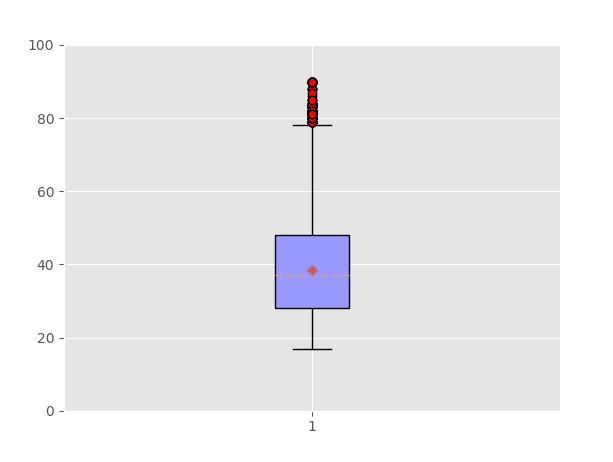3.whis参数
上文提到，我们的上下界用到了1.5倍的四分位值去定义。
如果whis=3时，上下界之外还出现异常值，我们称其为极端异常值。

展开全文python
• 今天小编就为大家分享一篇使用pandas的box_plot去除异常值，具有很好的参考价值，希望对大家有所帮助。一起跟随小编过来看看吧
• ## python用箱型图进行异常值检测

万次阅读 多人点赞 2016-05-16 20:52:01
异常值检测：数据挖掘工作中的第一步就是异常值检测，异常值的存在会影响实验结果。异常值是指样本中的个别值，也称为离群点，其数值明显偏离其余的观测值。常用检测方法3σ\sigma原则和箱型图。其中，3σ\sigma原则...数据挖掘
• 代码到这为止,就已经可以显示带有异常值箱型图了,但为了标注出异常值的数值,还需要以下代码进行标注. x = p['fliers'].get_xdata()# 'flies'即为异常值的标签 y = p['fliers'].get_ydata() y.sort() #用...python
• R语言-使用箱型图进行数据异常值分析 首先介绍本文章的主要内容： 1.检查批量数据的完整性（录入不全） 2.异常值分析（录入错误以及数据不合理） 箱形图（英文：Box plot），是一种用作显示一组数据分散情况资料的...大数据 r语言
• 异常值是用1.5IQR来推测的，IQR=Q3-Q1，是25%-75%之间的数值， Q4-Q3理论上应该只有0.5个IQR，超过1.5个IQR，则说明数据比较异常了。 上限是非异常范围内的最大值。下限是非异常范围内的最小值。 首先要知道...
• 如下：image.png中间的两个数是12和14，平均数13即为中位数。14以上的数字，最中间的数字是20即为上四分位数。12以下中间的数字是4即为下四分位数。当然，也是更严谨的计算方法。对样本数据或者全部数据线性回归，...
• 简述： ...也可以往盒里面加入平均(mean)。如下。下四分位数、中位数、上四分位数组成一个“带有隔间的盒子”。上四分位数到最大之间建立一条延伸线，这个延伸线成为“胡须(whisker)”。 ...机器学习 人工智能
• 箱线图四分位检测异常值(Box Plot Diagram to Identify Outliers)，箱型图提供了识别异常值的一个标准，即异常值通常被定义为 小于QL-1.5IQR 或大于 QU+1.5IQR 的值。箱线图识别异常值离群值是数据序列中的一个极端...
• type='dict') #画箱线图，直接使用DataFrame的方法 x = p['fliers'].get_xdata() # 'flies'即为异常值的标签 y = p['fliers'].get_ydata() y.sort() #从小到大排序，该方法直接改变原对象 #用annotate添加注释...
•Python 数据预处理
• 在数据处理中，线图常用来检测异常值。 数据文件data01.xls 1.绘制线图使用boxplot()函数 import pandas as pd import matplotlib.pyplot as plt # 读取excel文件 file_01 = pd.read_excel("data01.xls")...python 机器学习 数据挖掘
• 常用检测方法3σ原则和箱型图。其中，3σ原则只适用服从正态分布的数据。在3σ原则下，异常值被定义为观察值和平均值的偏差超过3倍标准差的值。P(|x−μ|>3σ)≤0.003，在正太分布假设下，大于3σ的值出现的概率小于...
• 最近有很多用户说到了年终需要回顾这一年的工作，根据这一年的数据看看有没有异常的情况，那么哪种能够清晰直观地展现出这一信息呢？答案只有一个，那就是....箱形最大的优点就是不受异常值的影响，能够准确稳定...
• 小白在之前文章中已经阐述了线图的原理，这里小白就把自己在工作中做的一个自动化异常值检测拿来分享一下~原理请看这篇博文（https://blog.csdn.net/Jarry_cm/article/details/95473014）小白根据线图的原理，...
• 更直观地展示这些数据，并且可以检测异常值的方法是使用箱型图 import pandas as pd catering_sale = 'catering_sale.xls' #餐饮数据 data = pd.read_excel(catering_sale, index_col = '日期' ) #...python
• 这种方法是利用线图的四分位距（IQR）对异常值进行检测，也叫Tukey`s test。其提供了识别异常值的一个标准：异常值通常被定义为小于QL-1.5IQR 或QU+1.5IQR。 QL：下四分位数，表示全部观察值中有四分之一的数据...算法 数据挖掘
• 线图概述线图又称箱形或盒须，主要从四分位数的角度来描述数据的分布。一个线图由上边缘线、上四分位数(QU)、中位数(me)，下四分位数(QL)和下边缘线组成。上、下四分位数之差称为四分位差，或内距或四分...
• 1. 箱型图分析 1、对数据没有作任何限制性要求（如服从某种特定的分布形式），它只是真实直观地表现数据分布的本来面貌； 2、判断异常值的标准以四分位数和四分位距为基础，四分位数具有一定的鲁棒性：多达25%的...
• 首先我们简单地区分一下离群点...箱型图 代码块 餐饮销售数据离群点检测代码： #-*- coding: utf-8 -*- import pandas as pd catering_sale = '../data/catering_sale.xls' #餐饮数据 data = pd.read_excel(ca
• 箱形最大的优点就是不受异常值的影响，能够准确稳定地描绘出数据的离散分布情况，同时也利于数据的清洗。我们一组序列数为例：12，15，17，19，20，23，25，28，30，33，34，35，36，37讲解1...
• 也可以往盒里面加入平均(mean)。如下。下四分位数、中位数、上四分位数组成一个“带有隔间的盒子”。上四分位数到最大之间建立一条延伸线，这个延伸线成为“胡须(whisker)”。 　由于现实数据中总是存在...boxplot 算法
• 异常值检验有很多种方法，这里主要说箱形。所谓的异常就是和大众不一样呗，就是指样本中出现的明显偏离大多数观测值的个别值。 线图(boxplot) 知识原理 （我手画了下，因为最开始是发在公众号上的，现在移图想...机器学习 人工智能
• 五数概念（five-number summary）由中位数（Q2Q2）、四分位数Q1Q1和Q3Q3、最大（Maximum）和最小（Minimum）观测组成，按次序Minimum，Q1Q1，Q2Q2，Q3Q3，Maximum。可疑的离群点通常是落在Q3Q3 之上或Q1Q1 之下......